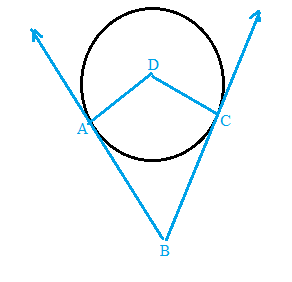Chapter 6.3, Problem 50EElementary Geometry For College St...

7th Edition
Alexander + 2 others
ISBN: 9781337614085

Solutions

Chapter
SectionElementary Geometry For College St...

7th Edition
Alexander + 2 others
ISBN: 9781337614085
Textbook Problem

The sides of ∠ A B C are tangent to ⊙ D at A and C, respectively. Explain why quadrilateral ABCD must be a kite.To determine

To explain:

Why quadrilateral ABCD must be a kite.

Explanation

Given that the sides of ABC are tangent to D at A and C, respectively.

To prove quadrilateral ABCD must be a kite.

The diagrammatic representation is given below,

Theorem:

The tangent segments to a circle from an external point are congruent.

Then AB¯andAC¯ are tangents to O from point A; B and C are points of tangency.

Therefore, AB¯AC¯

Also DA and DC are equal since DA and DC are radius of the circle (radius is same for all points on the circle)

Still sussing out bartleby?

Check out a sample textbook solution.

See a sample solution

The Solution to Your Study Problems

Bartleby provides explanations to thousands of textbook problems written by our experts, many with advanced degrees!

Get Started

Define a Type I error and a Type Il error and explain the consequences of each.

Statistics for The Behavioral Sciences (MindTap Course List)

In Exercises 21-24, find the distance between the given points. 21. (1, 3) and (4, 7)

Applied Calculus for the Managerial, Life, and Social Sciences: A Brief Approach

Convert the expressions in Exercises 6584 to power form. x2y

Finite Mathematics and Applied Calculus (MindTap Course List)

0 1 ∞

Study Guide for Stewart's Multivariable Calculus, 8th